# Fundamental system of solutions

(diff) ← Older revision | Latest revision (diff) | Newer revision → (diff)

of a linear homogeneous system of ordinary differential equations

A basis of the vector space of real (complex) solutions of that system. (The system may also consist of a single equation.) In more detail, this definition can be formulated as follows.

A set of real (complex) solutions(given on some set) of a linear homogeneous system of ordinary differential equations is called a fundamental system of solutions of that system of equations (on) if the following two conditions are both satisfied: 1) if the real (complex) numbersare such that the functionis identically zero on, then all the numbers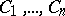are zero; 2) for every real (complex) solution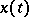of the system in question there are real (complex) numbers(not depending on) such thatIfis an arbitrary non-singular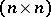-dimensional matrix, and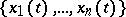is a fundamental system of solutions, then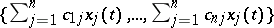is also a fundamental system of solutions; every fundamental system of solutions can be obtained by such a transformation from a given one.

If a system of differential equations has the form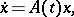(1)

where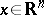(or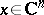), if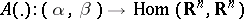and if the mappingis summable on every segment contained in(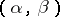is a bounded or unbounded interval in), then the vector space of solutions of this system is isomorphic to(respectively,). Consequently, the system (1) has an infinite set of fundamental systems of solutions, and each such fundamental system consists ofsolutions. For example, for the system of equations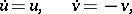an arbitrary fundamental system of solutions has the form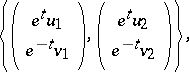where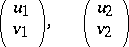are arbitrary linearly independent column vectors.

Every fundamental system of solutions of (1) has the form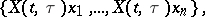whereis the Cauchy operator of (1),is an arbitrary fixed number in, andis an arbitrary fixed basis of(respectively,).

If the system of differential equations consists of the single equation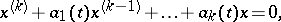(2)

where the functions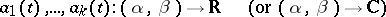are summable on every segment contained in(is a bounded or unbounded interval in), then the vector space of solutions of this equation is isomorphic to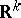(respectively,). Consequently, the equation (2) has infinitely many fundamental sets of solutions, and each of them consists ofsolutions. For example, the equationhas fundamental system of solutions; the general real solution of this equation is given by the formula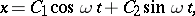where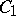andare arbitrary real constants.

If a system of differential equations has the form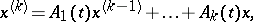(3)

where(or) and if for all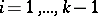the mappings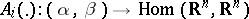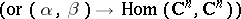are summable on every segment contained in(whereis a bounded or unbounded interval in), then the space of solutions of this system is isomorphic to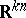(respectively,); there are fundamental systems of solutions of (3), and each of them consists of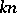solutions.

For linear homogeneous systems of differential equations that are not solved with respect to their leading derivatives, even if the coefficients of the system are constant, the number of solutions that appear in a fundamental system of solutions (that is, the dimension of the vector space of solutions) cannot always be calculated as easily as in the cases above. (In , Sect. 11 there is an examination of such a calculation for linear systems of differential equations with constant coefficients that are not solved with respect to their leading derivatives.)

How to Cite This Entry:
Fundamental system of solutions. Encyclopedia of Mathematics. URL: http://encyclopediaofmath.org/index.php?title=Fundamental_system_of_solutions&oldid=14876
This article was adapted from an original article by V.M. Millionshchikov (originator), which appeared in Encyclopedia of Mathematics - ISBN 1402006098. See original article